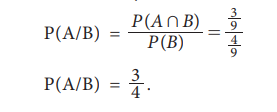Home | | Statistics 11th std | Conditional Probability

# Conditional Probability

Consider the following situations: i. two events occur successively or one after the other ii. both event A and event B occur together.

Conditional Probability

Consider the following situations:

i. two events occur successively or one after the other (e.g) A occurs after B has occurred and

ii. both event A and event B occur together.

### Example 8.13

There are 4000 people living in a village including 1500 female. Among the people in the village, the age of 1000 people is above 25 years which includes 400 female. Suppose a person is chosen and you are told that the chosen person is a female. What is the probability that her age is above 25 years?

### Solution:

Here, the event of interest is selecting a female with age above 25 years. In connection with the occurrence of this event, the following two events must happen.

A:a person selected is female

B:a person chosen is above 25 years.

### Situation1:

We are interested in the event B, given that A has occurred. This event can be denoted by B|A. It can be read as ŌĆ£B given AŌĆØ. It means that first the event A occurs then under that condition, B occurs. Here, we want to find the probability for the occurrence of B|A i.e., P(B|A). This probability is called conditional probability. In reverse, the probability for selecting a female given that a person has been selected with age above 25 years is denoted by P(A|B).

### Situation 2:

Suppose that it is interested to select a person who is both female and with age above 25 years. This event can be denoted by A Ōł®B.

Calculation of probabilities in these situations warrant us to have another theorem namely Multiplication theorem. It is derived based on the definition of conditional probability.## Definition of Conditional of Probability

If P(B) > 0, the conditional probability of A given B is defined asIf P(B) = 0, then P(AŌł®B) = 0. Hence, the above formula is meaningless when P(B) = 0. Therefore, the conditional probability P(A|B) can be calculated only when P(B)>0.

The need for the computation of conditional probability is described in the following illustration.

Illustration

A family is selected at random from the set of all families in a town with one twin pair. The sample space is

S = {(boy, boy), (boy, girl), (girl, boy), (girl, girl)}.

Define the events

A: the randomly selected family has two boys, and

B: the randomly selected family has a boy.

Let us assume that all the families with one twin pair are equally likely. Since

A = {(boy, boy)},

B = {(boy, boy), (boy, girl), (girl, boy)},

AŌł®B = A = {(boy, boy)}.

Applying the classical definition of probability, it can be calculated thatSuppose that the randomly selected family has a boy. Then, the probability that the other child in the pair is a girl can be calculated using conditional probability as### Example 8.14

A number is selected randomly from the digits11 through 19. Consider the events

A = { 11,14, 16, 18, 19 }

B = { 12, 14, 18, 19 }

C = { 13, 15, 18, 19 }.

Find (i) P(A/B) (ii) P(A/C) (iii) P(B/C) (iv) P (B/A)

### Solution:Therefore, the probability for the occurrence of A given that B has occurred isThe probability for the occurrence of A given that C has occurred isSimilarly, the conditional probability of B given C isand the conditional probability of B given A is### Example 8.15

A pair of dice is rolled and the faces are noted. Let

A: sum of the faces is odd, B: sum of the faces exceeds 8, and

C: the faces are different then find (i) P (A/C) (ii) P (B/C)

### Solution:

The outcomes favourable to the occurrence of these events are

A = { (1,2), (1,4), (1,6), (2,1), (2,3), (2,5), (3,2), (3,4), (3,6), (4,1), (4,3), (4,5), (5,2), (5,4), (5,6), (6,1), (6,3), (6,5) }

B = { (3,6), (4,5), (4,6), (5,4), (5,5), (5,6), (6,3), (6,4), (6,5), (6,6) }

C = { (1,2), (1,3), (1,4), (1,5), (1,6), (2,1), (2,3), (2,4), (2,5), (2,6), (3,1), (3,2), (3,4), (3,5), (3,6), (4,1), (4,2), (4,3), (4,5), (4,6),  (5,1), (5,2), (5,3), (5,4), (5,6), (6,1), (6,2), (6,3), (6,4), (6,5) }

Since A and B are proper subsets of C, AŌł®C = A and BŌł®C = B.Hence, the probability for the sum of the faces is an odd number given that the faces are different isSimilarly, the probability for the sum of the faces exceeds 8 given that the faces are different is## Axioms

The conditional probabilities also satisfy the same axioms introduced in Section 8.3.

If S is the sample space of a random experiment and B is an event in the experiment, then

(i) P(A/B) Ōēź 0 for any event A of S.

(ii) P(S/B) = 1

(iii) If A1, A2, ŌĆ” is a sequence of mutually exclusive events, thenIn continuation of conditional probability, another property of events, viz., independence can be studied. It is discussed in the next section. Also, multiplication theorem, a consequence of conditional probability, will be studied later.

Study Material, Lecturing Notes, Assignment, Reference, Wiki description explanation, brief detail
11th Statistics : Chapter 8 : Elementary Probability Theory : Conditional Probability |